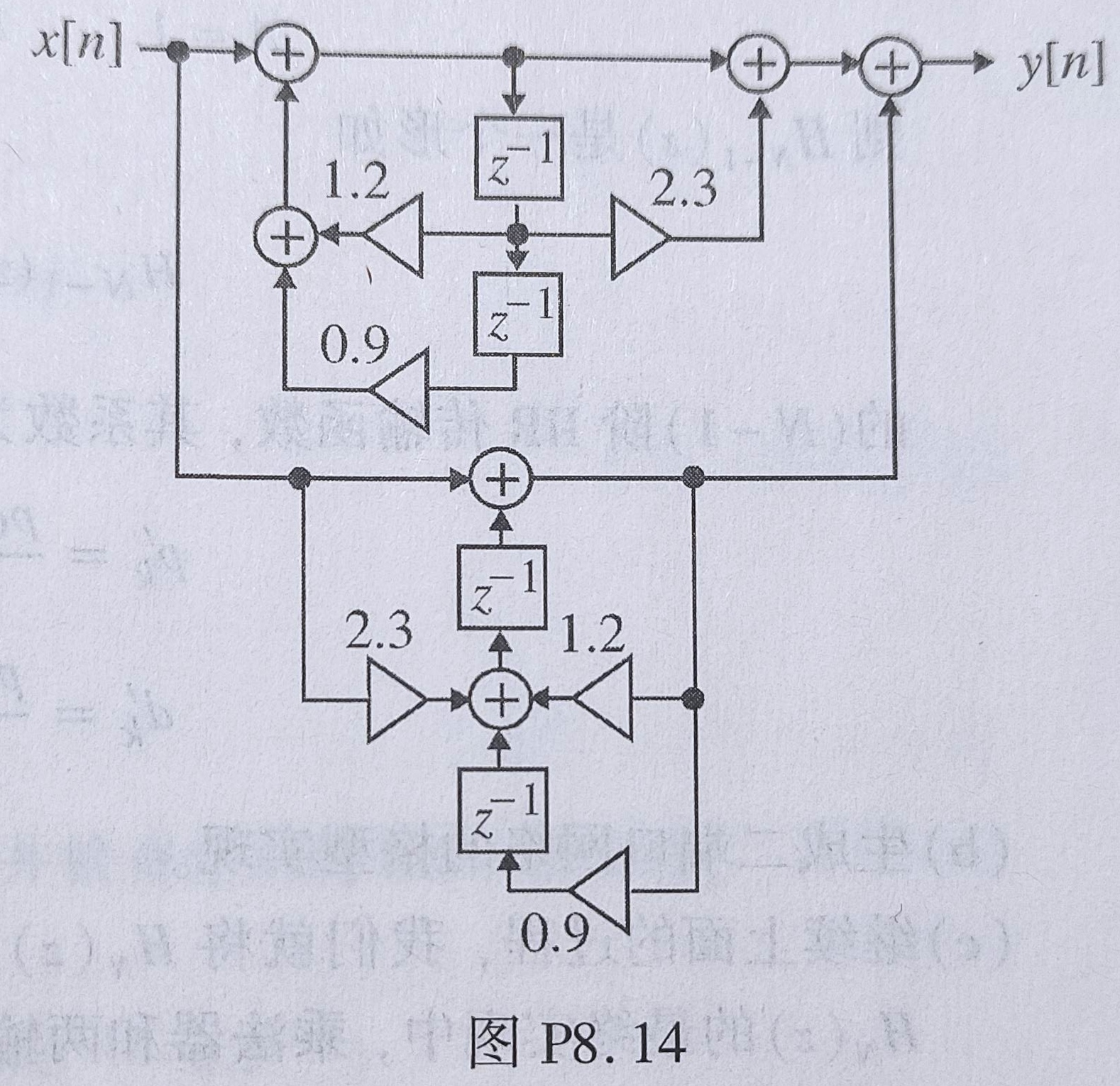# 结构单元

## 等效结构

1. 将所有箭头反转
2. 把节点换成加法器，加法器换成节点
3. 交换输入和输出

1. 所需的存储单元和乘法次数不同，前者影响复杂度，后者影响运算速度
2. 有限精度下，不同结构的误差和稳定性不同

# 系统的基本实现

## 串联实现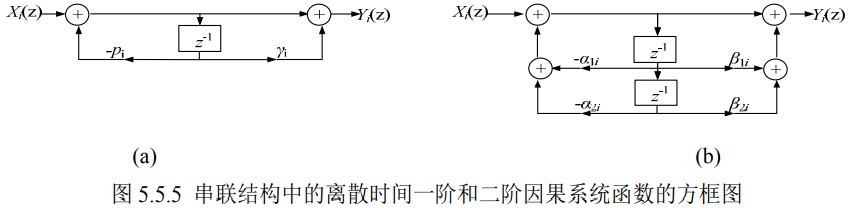## 并联实现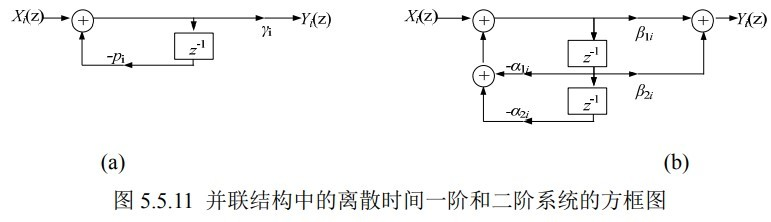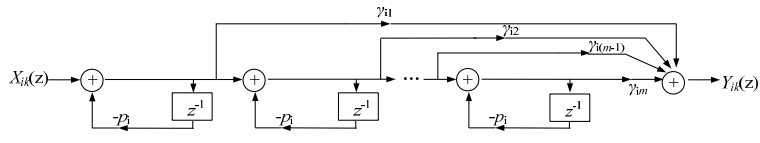## FIR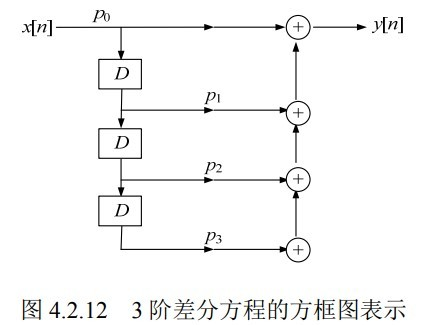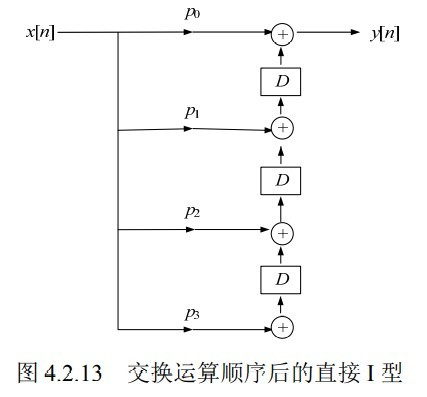## IIR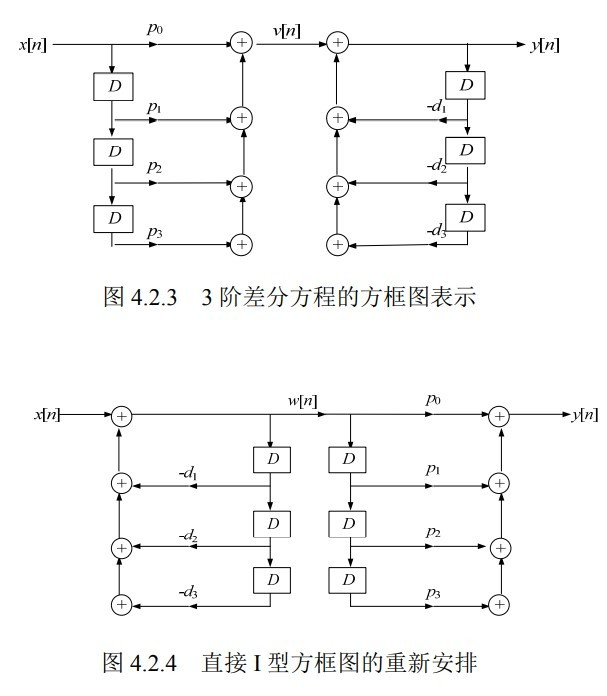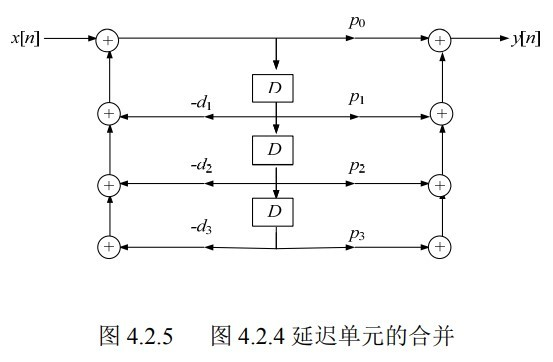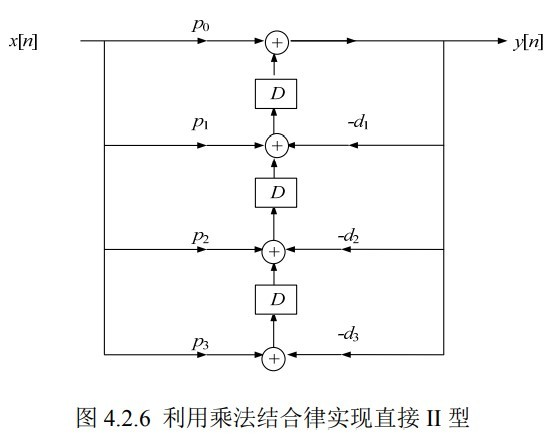直接Ⅰ型 直接Ⅱ型

# 习题

8.28 将下面三个因果一阶 LTI 系统级联：
$$H_1(z)=\frac{1-0.6z^{-1}}{1+0.25z^{-1}}\\ H_2(z)=\frac{0.2+z^{-1}}{1+0.3z^{-1}}\\ H_3(z)=\frac{2}{1+0.25z^{-1}}$$
（a）求整个系统的传输函数
（b）求差分方程
（c）每节用直接Ⅱ型，求系统实现
（d）求并联Ⅰ型实现
（e）求冲激响应

$$H(z)=H_1(z)H_2(z)H_3(z)=\frac{0.4+1.76z^{-1}-1.2z^{-2}}{1+0.8 z^{-1}+0.2125 z^{-2}+0.0187z^{-3}}\\ y[n]+0.8 y[n-1]+0.2125 y[n-2]+0.0187y[n-3]= 0.4x[n] +1.76 x[n-1]-1.2x[n-2]$$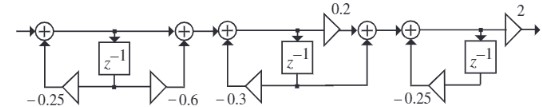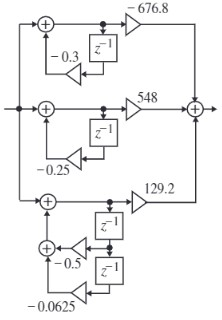$$h[n]=-676.8(-0.3)^n u[n]+548(-0.25)^n u[n]+129.2 129.2(n+1)(-0.25)^{(n+1)}u[n]$$

8.32 下面这玩意是不是典范结构，不是的话就改成典范结构。